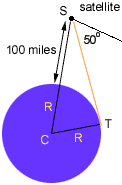Name: Jessica Who is asking: Student Level: All Question: I'm struggling with this problem - any advice would be greatly appreciated. Thanks! A satellite is orbiting the earth at an altitude of 100 miles. If the angle of depression from the satellite to the horizon is 50 degrees, what is the radius (to the nearest mile) of the planet? Thanks again, Jessica Hi Jessica, In the diagram below C is the center of the planet, R is the radius of the planet, S is the position of the satellite and the line ST is tangent to the planet.Since the angle of depression from the satellite to the horizon is 50o, angle TSC is 40o. Thus, since CST is a right angle, sin (40o) = R/100 + R Hence (100 +R) sin (40o) = R and thus 100 sin (40o) = R (1 - sin (40o)) But sin (40o) = 0.643 and thus R = 64.3/(1 - 0.643) = 180.1 miles Cheers, Harley Go to Math Central